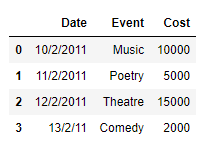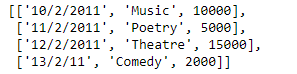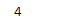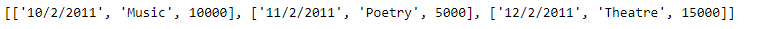# Create a list from rows in Pandas dataframe

Python list is easy to work with and also list has a lot of in-built functions to do a whole lot of operations on lists. Pandas dataframe’s columns consist of series but unlike the columns, Pandas dataframe rows are not having any similar association. In this post, we are going to discuss several ways in which we can extract the whole row of the dataframe at a time.

Solution #1: In order to iterate over the rows of the Pandas dataframe we can use `DataFrame.iterrows()` function and then we can append the data of each row to the end of the list.

 `# importing pandas as pd ` `import` `pandas as pd ` ` `  `# Create the dataframe ` `df ``=` `pd.DataFrame({``'Date'``:[``'10/2/2011'``, ``'11/2/2011'``, ``'12/2/2011'``, ``'13/2/11'``], ` `                    ``'Event'``:[``'Music'``, ``'Poetry'``, ``'Theatre'``, ``'Comedy'``], ` `                    ``'Cost'``:[``10000``, ``5000``, ``15000``, ``2000``]}) ` ` `  `# Print the dataframe ` `print``(df) `

Output :Now we will use the `DataFrame.iterrows()` function to iterate over each of the row of the given Dataframe and construct a list out of the data of each row.

 `# Create an empty list ` `Row_list ``=``[] ` ` `  `# Iterate over each row ` `for` `index, rows ``in` `df.iterrows(): ` `    ``# Create list for the current row ` `    ``my_list ``=``[rows.Date, rows.Event, rows.Cost] ` `     `  `    ``# append the list to the final list ` `    ``Row_list.append(my_list) ` ` `  `# Print the list ` `print``(Row_list) `

Output :As we can see in the output, we have successfully extracted each row of the given dataframe into a list. Just like any other Python’s list we can perform any list operation on the extracted list.

 `# Find the length of the newly  ` `# created list ` `print``(``len``(Row_list)) ` ` `  `# Print the first 3 elements ` `print``(Row_list[:``3``]) `

Output :Solution #2: In order to iterate over the rows of the Pandas dataframe we can use `DataFrame.itertuples()` function and then we can append the data of each row to the end of the list.

 `# importing pandas as pd ` `import` `pandas as pd ` ` `  `# Create the dataframe ` `df ``=` `pd.DataFrame({``'Date'``:[``'10/2/2011'``, ``'11/2/2011'``, ``'12/2/2011'``, ``'13/2/11'``], ` `                    ``'Event'``:[``'Music'``, ``'Poetry'``, ``'Theatre'``, ``'Comedy'``], ` `                    ``'Cost'``:[``10000``, ``5000``, ``15000``, ``2000``]}) ` ` `  `# Print the dataframe ` `print``(df) `

Output :Now we will use the `DataFrame.itertuples()` function to iterate over each of the row of the given Dataframe and construct a list out of the data of each row.

 `# Create an empty list ` `Row_list ``=``[] ` ` `  `# Iterate over each row ` `for` `rows ``in` `df.itertuples(): ` `    ``# Create list for the current row ` `    ``my_list ``=``[rows.Date, rows.Event, rows.Cost] ` `     `  `    ``# append the list to the final list ` `    ``Row_list.append(my_list) ` ` `  `# Print the list ` `print``(Row_list) `

Output :As we can see in the output, we have successfully extracted each row of the given dataframe into a list. Just like any other Python’s list we can perform any list operation on the extracted list.

 `# Find the length of the newly  ` `# created list ` `print``(``len``(Row_list)) ` ` `  `# Print the first 3 elements ` `print``(Row_list[:``3``]) `

Output :My Personal Notes arrow_drop_upCheck out this Author's contributed articles.

If you like GeeksforGeeks and would like to contribute, you can also write an article using contribute.geeksforgeeks.org or mail your article to contribute@geeksforgeeks.org. See your article appearing on the GeeksforGeeks main page and help other Geeks.

Please Improve this article if you find anything incorrect by clicking on the "Improve Article" button below.

Article Tags :

Be the First to upvote.

Please write to us at contribute@geeksforgeeks.org to report any issue with the above content.